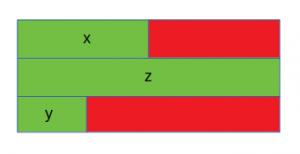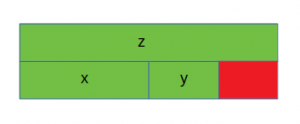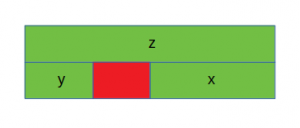# Is sizeof for a struct equal to the sum of sizeof of each member?

## Is sizeof for a struct equal to the sum of sizeof of each member?

The sizeof for a struct is not always equal to the sum of sizeof of each individual member. This is because of the padding added by the compiler to avoid alignment issues. Padding is only added when a structure member is followed by a member with a larger size or at the end of the structure.

Different compilers might have different alignment constraints as C standards state that alignment of structure totally depends on the implementation.

Take a step-up from those “Hello World” programs. Learn to implement data structures like Heap, Stacks, Linked List and many more!

Let’s take a look at the following examples for better understanding:

• Case 1:
 `// C program to illustrate` `// size of struct` `#include ` ` ` `int` `main()` `{` ` ` `    ``struct` `A {` ` ` `        ``// sizeof(int) = 4` `        ``int` `x;` `        ``// Padding of 4 bytes` ` ` `        ``// sizeof(double) = 8` `        ``double` `z;` ` ` `        ``// sizeof(short int) = 2` `        ``short` `int` `y;` `        ``// Padding of 6 bytes` `    ``};` ` ` `    ``printf``(``"Size of struct: %ld"``, ``sizeof``(``struct` `A));` ` ` `    ``return` `0;` `}`

Output:

```Size of struct: 24
```The red portion represents the padding added for data alignment and the green portion represents the struct members. In this case, x (int) is followed by z (double), which is larger in size as compared to x. Hence padding is added after x. Also, padding is needed at the end for data alignment.

• Case 2:
 `// C program to illustrate` `// size of struct` `#include ` ` ` `int` `main()` `{` ` ` `    ``struct` `B {` `        ``// sizeof(double) = 8` `        ``double` `z;` ` ` `        ``// sizeof(int) = 4` `        ``int` `x;` ` ` `        ``// sizeof(short int) = 2` `        ``short` `int` `y;` `        ``// Padding of 2 bytes` `    ``};` ` ` `    ``printf``(``"Size of struct: %ld"``, ``sizeof``(``struct` `B));` ` ` `    ``return` `0;` `}`

Output:

```Size of struct: 16
```In this case, the members of the structure are sorted in decreasing order of their sizes. Hence padding is required only at the end.

• Case 3:
 `// C program to illustrate` `// size of struct` `#include ` ` ` `int` `main()` `{` ` ` `    ``struct` `C {` `        ``// sizeof(double) = 8` `        ``double` `z;` ` ` `        ``// sizeof(short int) = 2` `        ``short` `int` `y;` `        ``// Padding of 2 bytes` ` ` `        ``// sizeof(int) = 4` `        ``int` `x;` `    ``};` ` ` `    ``printf``(``"Size of struct: %ld"``, ``sizeof``(``struct` `C));` ` ` `    ``return` `0;` `}`

Output:

```Size of struct: 16
```In this case, y (short int) is followed by x (int) and hence padding is required after y. No padding is needed at the end in this case for data alignment.

C language doesn’t allow the compilers to reorder the struct members to reduce the amount of padding. In order to minimize the amount of padding, the struct members must be sorted in a descending order (similar to the case 2).

Last Updated on November 13, 2021 by admin

## Array of Strings in C++Array of Strings in C++

Array of Strings in C++ (All Different Ways to Create) In C and C++, a

## How to assign values to variables in C++How to assign values to variables in C++

How to assign values to variables in Python and other languages This article discusses methods

## Program to print all the numbers divisible by 3 and 5 for a given numberProgram to print all the numbers divisible by 3 and 5 for a given number

Program to print all the numbers divisible by 3 and 5 for a given number

## Arrays in C/C++Arrays in C/C++

Arrays in C/C++ An array in C/C++ or be it in any programming language is

## Generating subarrays using recursionGenerating subarrays using recursion

Generating subarrays using recursion Given an array, generate all the possible subarrays of the given

## Program to print the diamond shapeProgram to print the diamond shape

Program to print the diamond shape Given a number n, write a program to print

## Program to calculate Electricity BillProgram to calculate Electricity Bill

Program to calculate Electricity Bill Given an integer U denoting the amount of KWh units of electricity

## Program to generate all possible valid IP addresses from given stringProgram to generate all possible valid IP addresses from given string

Program to generate all possible valid IP addresses from given string Given a string containing